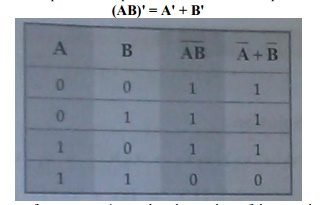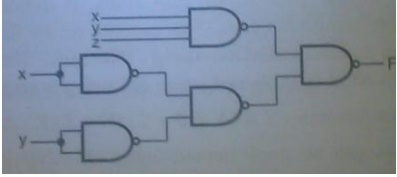Home | | Digital Electronics | Important Short Questions and Answers: Minimization Techniques and Logic Gates

# Important Short Questions and Answers: Minimization Techniques and Logic Gates

Digital Electronics - Minimization Techniques and Logic Gates - Important Short Questions and Answers: Minimization Techniques and Logic Gates

MINIMIZATION TECHNIQUES AND LOGIC GATES

1.         State DemorganŌĆÖs Theorem.

De Morgan suggested two theorems that form important part of Boolean algebra. They are,

1) The complement of a product is equal to the sum of the complements.

(AB)' = A' + B'2) The complement of a sum term is equal to the product of the complements.

(A + B)' = A'B'2.    Draw an active-high tri-state buffer and write its truth table.3.         What is a totem pole output?

Totem pole output is a standard output of a TTL gate. It is specifically designed to reduce the propagation delay in the circuit and to provide sufficient output power for high fan-out.

4.    Draw the TTL Inverter (NOT) Circuit.5.    Implement using NAND gates only, F=xyz+xŌĆ▓yŌĆ▓6.           What are DonŌĆÖt care terms?

In some logic circuits certain input conditions never occur, therefore the corresponding output never appears. In such cases the output level is not defined, it can be either high or low. These output levels are indicated by ŌĆśXŌĆÖ or ŌĆśdŌĆÖ in the truth tables and are called donŌĆÖt care conditions or incompletely specified functions.

7.           Apply De-MorganŌĆÖs theorem to [(A+B)+C] ŌĆ▓.

Given [(A+B)+C] ŌĆ▓= (A+B) ŌĆ▓.CŌĆ▓

= (AŌĆ▓.BŌĆ▓).CŌĆ▓

[(A+B)+C] ŌĆ▓ = AŌĆ▓BŌĆ▓CŌĆ▓

8.           Convert 0.35 to equivalent hexadecimal number.

Given (0.35)10 =0.35 x 16=5.60 =0.60 x 16=9.60 =0.60 x 16=9.60

(0.35)10 =(0.599)16

9.           Convert Y=A+BCŌĆ▓+AB+AŌĆ▓BC into canonical form.

Given Y=A+BCŌĆ▓+AB+AŌĆ▓BC Y=A(B+BŌĆ▓)(C+CŌĆ▓)+(A+AŌĆ▓)BCŌĆ▓+AB(C+CŌĆ▓)+AŌĆ▓BC Y=ABC+ABCŌĆ▓+ABŌĆ▓C+ABŌĆ▓CŌĆ▓+ABCŌĆ▓+AŌĆ▓BCŌĆ▓+ABC+ABCŌĆ▓+AŌĆ▓BC

Y=ABC+ABCŌĆ▓+ABŌĆ▓C+ABŌĆ▓CŌĆ▓+AŌĆ▓BCŌĆ▓+AŌĆ▓BC

10.    State the advantages of CMOS logic.

┬Ę              Consumes less power.

┬Ę              Can be operated at high voltages, resulting in improved noise immunity.

┬Ę              Fan-out is more.

┬Ę              Better noise margin.

11.    Define ŌĆśmin termŌĆÖ and ŌĆśmax termŌĆÖ.

(i)          A  product  term  containing  all  the  variables  of  the  function  in  either complemented or uncomplemented form is called a min term.

(ii) A sum term containing all the variables of the function in either complemented or uncomplemented form is called a max term.

12.    Write a note on tri-state gates.

It is a digital circuit that exhibits three states. Two of the states are signals equivalent to logic1 and logic 0. The third state is high impedance state. High impedance state behaves like a open circuit.13.      Prove that the logical sum of all min terms of a Boolean function of 2 variables is 1.

Consider  two  variables  as  A and  B.  For  two  variables  A and  B  minterms  are:

AŌĆ▓BŌĆ▓,AŌĆ▓B,ABŌĆ▓,AB. The logical sum of these minterms are given by

F= AŌĆ▓BŌĆ▓+AŌĆ▓B+ABŌĆ▓+AB

= AŌĆ▓(BŌĆ▓+B)+A(BŌĆ▓+B)                 (BŌĆ▓+B=1)

= AŌĆ▓(1)+A(1)                             (AŌĆ▓+A=1)

F=1

Hence it is to be proved.

14.    Show that a positive logic NAND gate is a negative logic NOR gate.Truth table for positive logic NAND gate and negative logic NOR gates are same and hence a positive logic NAND gate is negative logic NOR gate.

15.    What is the significance of high impedance state in tri-state gates?

┬Ę              High impedance state of a three-state gate provides a special feature not available in other gates.

┬Ę              Because of this features a larger number of three state gate output can be connected with wires to form a common line without endangering loading effects.

16.    Simplify the following Boolean Expression to a minimum number of literals.

(BCŌĆ▓+AŌĆ▓D)(ABŌĆ▓+CDŌĆ▓)

F=(BCŌĆ▓+AŌĆ▓D)(ABŌĆ▓+CDŌĆ▓)

=BCŌĆ▓ABŌĆ▓+BCŌĆ▓CDŌĆ▓+AŌĆ▓DABŌĆ▓+AŌĆ▓DCDŌĆ▓ (A.AŌĆ▓=0)

= AB BŌĆ▓CŌĆ▓+BCCŌĆ▓DŌĆ▓+AAŌĆ▓ BŌĆ▓D+AŌĆ▓CDDŌĆ▓

F=0

17.    Define the term Fan out.

It is the maximum number of inputs which have same family that the gate can drive maintaining its output within the specified limits.

18.    Simplify the given Boolean Expression F=xŌĆ▓+xy+xzŌĆ▓+xyŌĆ▓zŌĆ▓.

F=xŌĆ▓+xy+xzŌĆ▓+xyŌĆ▓zŌĆ▓

= xŌĆ▓+x(y+zŌĆ▓+yŌĆ▓zŌĆ▓)                    (A+AŌĆ▓B=A+B)

= xŌĆ▓+y+zŌĆ▓+yŌĆ▓zŌĆ▓

= xŌĆ▓+y+zŌĆ▓(1+yŌĆ▓)                      (1+AŌĆ▓=1)

F = xŌĆ▓+y+zŌĆ▓

19.    Implement the given function using NAND gates F(x,y,z)= ╬Żm(0,6).

F(x,y,z)=xŌĆ▓yŌĆ▓zŌĆ▓+xyzŌĆ▓20.    State Distributive Law.

Distributive law of dot(.) over plus(+) is given by a.(b+c) = a.b + a.c

Distributive law of plus(+) over dot(.) is given by a+b.c = (a+b).(a+c)

21.    What is Prime Implicant?

A prime implicant is a group of minterms which cannot be combined with any other minterms or groups.

22.      Simplify the following Boolean expression into one literal. WŌĆ▓X(ZŌĆ▓+YZ)+X(W+ YŌĆ▓Z)

F= WŌĆ▓X(ZŌĆ▓+YZ)+X(W+ YŌĆ▓Z)

=             WŌĆ▓XZŌĆ▓+WŌĆ▓XYZ+WX+XYŌĆ▓Z

=X(WŌĆ▓ZŌĆ▓+WŌĆ▓YZ+W+YŌĆ▓Z)

=             X(WŌĆ▓ZŌĆ▓+W+Z(YŌĆ▓+WŌĆ▓Y))

=             X(WŌĆ▓ZŌĆ▓+W+Z(YŌĆ▓+ Y )( YŌĆ▓+WŌĆ▓))

=             X(WŌĆ▓ZŌĆ▓+W+Z( YŌĆ▓+WŌĆ▓))

=             X(WŌĆ▓ZŌĆ▓+W+ZYŌĆ▓+WŌĆ▓Z)

=             X(WŌĆ▓(ZŌĆ▓+Z)+W+ZYŌĆ▓)

=             X(WŌĆ▓+W+ZYŌĆ▓)

=             X(1+ZYŌĆ▓)=X.1

F            =X

23.    Draw the CMOS inverter circuit.Study Material, Lecturing Notes, Assignment, Reference, Wiki description explanation, brief detail
Digital Electronics : Minimization Techniques and Logic Gates : Important Short Questions and Answers: Minimization Techniques and Logic Gates |